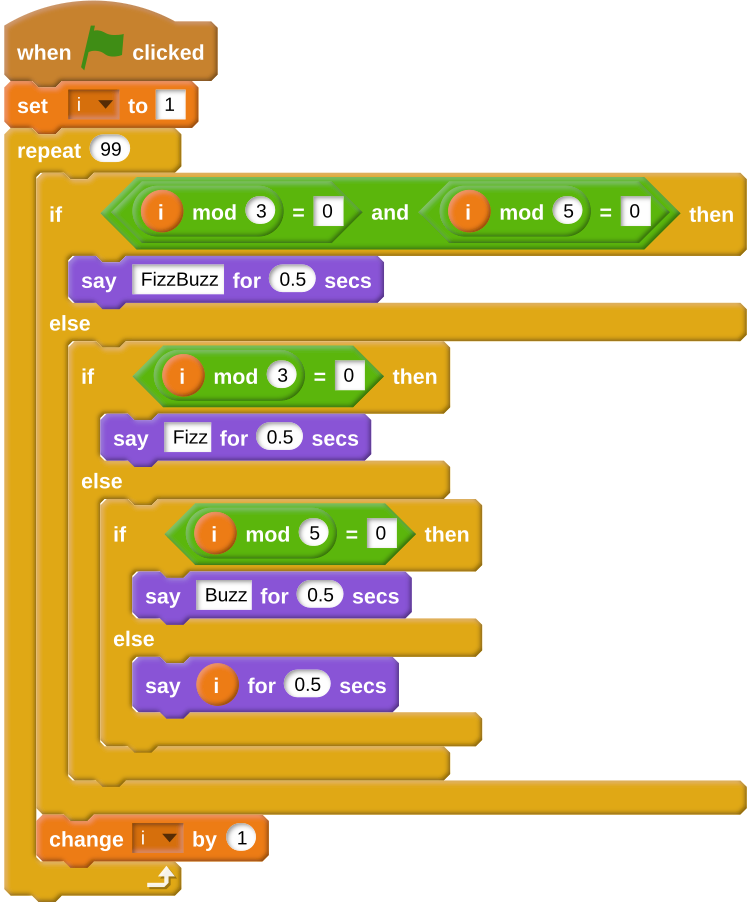## Want to keep learning?

This content is taken from the Raspberry Pi Foundation & National Centre for Computing Education's online course, Scratch to Python: Moving from Block- to Text-based Programming. Join the course to learn more.
3.4

## Raspberry Pi Foundation# Introducing pseudocode

Although showing learners direct translations between block-based and text-based languages can be useful, this is not always possible. For instance, most block-based languages cannot communicate with a computer’s operating system, so file handling isn’t really possible, nor are communication between one computer and another, or access to peripheral input and output devices.

Pseudocode is a way of expressing an algorithm without conforming to specific syntactic rules. By learning to read and write pseudocode, learners can more easily communicate ideas and concepts to other programmers, even though they may be using completely different languages. What’s more, algorithmic solutions to many problems are often provided in pseudocode on sites such as StackOverflow and Wikipedia, meaning an ability to translate between pseudocode and a given programming language is a valuable skill.

There is no such thing as correct pseudocode, although there are a few generally accepted notations that are widely understood by programmers (e.g. x <-- 10 to create a variable called x with a value of 10), no matter which language they use.

Let’s have a look at a simple pseudocode example and see its equivalent scripts in both Scratch and Python.

## FizzBuzz

FizzBuzz is a common programming challenge with hundreds of different solutions in many languages. The challenge is as follows.

For every number from 1 to 100, output Fizz if the number is divisible by 3, output Buzz if the number is divisible by 5, and output FizzBuzz if the number is divisible by both 3 and 5. If none of these conditions match, then just output the number.

Let’s have a look at one possible pseudocode interpretation of this challenge:

FOR i <-- 1 TO 100 DO
IF i is divisible by 3 AND i is divisible by 5 THEN
OUTPUT "FizzBuzz"
ELSE IF i is divisible by 3 THEN
OUTPUT "Fizz"
ELSE IF i is divisible by 5 THEN
OUTPUT "Buzz"
ELSE
OUTPUT i


Here, you start by using a loop to iterate over all the numbers. Then IF, ELSE IF, and ELSE control statements are used to decide the output depending on the number.

This code can easily be translated into any programming language.

Here’s the same algorithm in Scratch:You might already begin to see how a program to address this type of problem can become overly complicated in a language like Scratch, where it is necessary to nest blocks within blocks and introduce pauses to enable the output to be clearly seen.

Here’s an example of a FizzBuzz algorithm in Python:

for i in range(1,100):
if i % 3 == 0 and i % 5 == 0:
print('FizzBuzz')
elif i % 3 == 0:
print('Fizz')
elif i % 5 == 0:
print('Buzz')
else:
print(i)


You’ll probably notice that this is much closer to the pseudocode. It is only one of many possible solutions to the problem, and in a text-based language your superpowers can be greatly expanded once you know a few tips and tricks.

For instance, here’s a version of the same program that’s a single line of code.

print("\n".join(["Fizz" * (i % 3 == 0) + "Buzz" *(i % 5 == 0) or str(i) for i in range(1, 100)]))


You don’t have to understand this code, but it provides an example of how efficient you can be with a text-based language once you have mastered some syntactic tricks.

## Try it out

Now it’s your turn to have a go at converting some pseudocode.

A prime number is one that is only divisible by the number 1 and itself. You can test if a number is prime by trying to divide it by every number from 2 onward.

Try translating this pseudocode into Python (you can translate it to Scratch first if you like):

number <-- 17
prime <-- TRUE

FOR i <-- 2 TO number
IF number is divisible by i THEN
prime <-- FALSE

IF prime = TRUE:
OUTPUT "prime"
ELSE
OUTPUT "not prime"


Check that you algorithm works by changing the value of number to various prime and non-prime numbers.

How did you get on? You could even try improving on your original algorithm to make it a little faster. Ask for help and share your solutions in the comments section below.